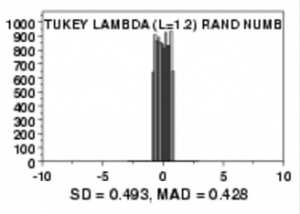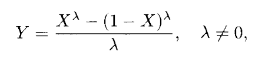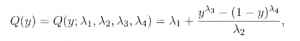# Tukey Lambda Distribution: Definition

Share on

Probability Distributions > Tukey Lambda Distribution

## What is the Tukey Lambda Distribution?The Tukey Lambda distribution (sometimes called the symmetric lambda distribution) has truncated tails.

The Tukey Lambda Distribution is a family of symmetric distributions with truncated (cut off) tails.

The distribution is defined numerically with three parameters:

Unlike most other probability distributions, there isn’t a “one size fits all” formula for the probability density function (PDF) and cumulative distribution function (CDF). You’ll usually see it defined in terms of quantiles or the following distribution function:The following image shows two vastly different shapes for λ values of 0.5 and 5.0:

So although there isn’t one general PDF for all possible values of λ, a PDF can easily be generated for specific values once you know the percentile function.

## GLD and the Percentile Function

The generalized lambda distribution is usually defined in terms of its four-parameter percentile function:.

Where:
0 ≤ y ≤ 1
and

• λ1, the location parameter,
• λ2, the scale parameter,
• λ3, skewness,
• λ4, kurtosis.

## Uses

Not surprisingly, the Tukey Lambda isn’t used for statistical modeling due to the lack of an easily definable PDF or CDF. It’s main use is to approximate other symmetric probability distributions such as:

It is also an exact match for some distributions, including:

## Origins

Tukey’s lambda distribution was first proposed by John Tukey in 1960 (although it was based on 1947 work by Hastings et. al). In the early 1970s the distribution was generalized by John Ramberg and Bruce Schmeiser for use in Monte Carlo simulations; Ramberg and colleagues went on to develop the curve-fitting< properties of the distribution in the late 70's. After you have fit the curve, you can then model the residuals from that curve. Curve fitting algorithms include: gradient descent, Gauss-Newton and the Levenberg–Marquardt algorithm.

## Tukey-Lambda PPCC Plot

One of the more common uses for the Tukey-Lambda distribution is in PPCC plot generation. A Tukey-Lambda PPCC plot (Probability Plot Correlation Coefficient Plot) is generated by software and is based on a set of inputted data. The plot results in a suggested model for the data.

References:
Tukey, J. (1960). The Practical Relationship Between the Common Transformations of Percentages of Counts and Amounts, Technical Report 36. Statistical Techniques Research Group, Princeton University.
Hastings, C. et. al (1947). Low moments for small samples: a comparative study of statistics. Annals of Mathematical Statistics, 18, 413-426.
Karian, Z. (2000). Fitting Statistical Distributions: The Generalized Lambda Distribution and Generalized Bootstrap Methods 1st Edition. Chapman and Hall/CRC
Ramberg, J & Schmeiser, B. (1972). An approximate method for generating symmetric random variables. Commun. ACM, 15:987-990.
Ramberg, J. et. al. (1979). A probability distribution and its uses in fitting data. Technometrics, 21(2):201-214, May 1979.

CITE THIS AS:
Stephanie Glen. "Tukey Lambda Distribution: Definition" From StatisticsHowTo.com: Elementary Statistics for the rest of us! https://www.statisticshowto.com/tukey-lambda-distribution/
---------------------------------------------------------------------------Need help with a homework or test question? With Chegg Study, you can get step-by-step solutions to your questions from an expert in the field. Your first 30 minutes with a Chegg tutor is free!